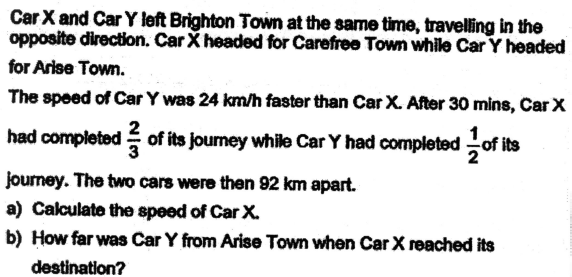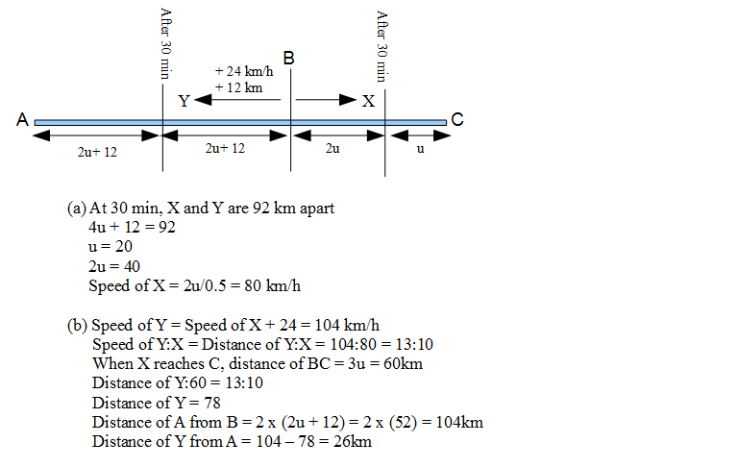# QuestionHi pls help to explain this . thank you.

Let the speed of Car X be 1u.

Carefree Town ——- Brighton Town ——- Arise Town

…………………… <——- Car X (1u, speed of Car X)

………………………………. Car Y (1u + 24km/h) ——->

speed of Car X + Car Y ——- 1u + 1u + 24 = 2u + 24

(2u + 24)/(1/2) = 92

(2u + 24) ——- 92 x 2 = 184

1u ——- (184 – 24)/2 = 80

(b)

2/3 ——- 80/2 = 40 (distance travelled by Car X in 30 min)

1/3 ——- 40/2 = 20

3/3 ——- 20 x 3 = 60

60/80 = 3/4 (h, time taken for Car X to reach Carefree Town)

80 + 24 = 104 (speed of Car Y)

92 – 40 or 104/2 = 52 (km, distance travelled by Car Y in 30min)

1/2 ——- 52

2/2 ——- 52 x 2 = 104

104 x (3/4) = 78 (km, distance travelled by Car Y in 3/4h)

104 – 78 = 26

Ans : (a) 80km/h; (b) 26km.

hi alfretztay, thank you.

Hi ATM 82517, don’t mention.

0 Replies 0 Likes

Since Y was 24 km/h faster, it would have travelled 12km more than X in 30 mins.

(92 – 12) ÷ 2 = 40

Car X had travelled 40 km in 0.5 hour,

whereas Car Y had travelled (40 + 12) = 52 km.

(a) Speed of Car X -> 40 ÷ 0.5 = 80 km/h

Car Y completed 1/2 of its journey after travelling 52km.

Arise Town was therefore, another 52 km away after 30 mins of travelling.

Car X completed 2/3 of its journey in 30 mins

To complete the remaining 1/3, it would take 30 ÷ 2 = 15 mins

Car Y, in 30 mins, travelled 52 km

Car Y, in 15 mins, would have travelled 52 ÷ 2 = 26 km

(b) Car Y would be 52 – 26 = 26 km away from Arise Town.

2 Replies 1 Like

Hi big devil, pardon me to ask – why divided by 2 ? thank you.

(92 – 12) ÷ 2 = 40

hi bigdevil, thank you so much.